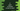# C program to print or format a number to words### C program to print a number in words :

In this C programming tutorial, we will learn how to format a number to words. The user will enter one number and our program will convert it to words. For example, if the user will enter 987, it will print Nine Eight Seven as output.

The algorithm we are going to use in this program is :

### Algorithm :

1. Ask the user to enter a number.
2. Read it and save it to an integer variable.
3. Convert the integer to an integer array.The array will hold the numbers in reverse order. For example, 345 number will become [5,4,3] array.
4. Next, scan the numbers in the array in reverse order. For example, the array [5,4,3] for the number 345, we will scan the number 3 first, 4 second and 5 third.
5. For the current scanned number, print out its value in words. For example, for 2, print Two.

### C program to print a number in words :

``````#include <stdio.h>
#include <stdlib.h>
#include <math.h>

int main()
{
//1
int num, i;

//2
printf("Enter a number : ");
scanf("%d", &num);

//3
int size = log10(num) + 1;
int *numArray = (int *)malloc(size * sizeof(int));

//4
for (i = 0; i < size; i++)
{
numArray[i] = num % 10;
num = num / 10;
}
//5
for (i = size - 1; i >= 0; i--)
{
//6
switch (numArray[i])
{
case 1:
printf("One ");
break;
case 2:
printf("Two ");
break;
case 3:
printf("Three ");
break;
case 4:
printf("Four ");
break;
case 5:
printf("Five ");
break;
case 6:
printf("Six ");
break;
case 7:
printf("Seven ");
break;
case 8:
printf("Eight ");
break;
case 9:
printf("Nine ");
break;
case 0:
printf("Zero ");
break;
}
}
//7
printf("\n");
}``````

### Explanation :

The commented numbers in the above program denote the step number below :

1. Create one integer num to hold the number and one integer i to use in the loop.
2. Ask the user to enter a number. Store that number in variable num.
3. Find the size of the number. First, we are finding out the log value of that number and then adding 1 to it. For example, log10(123) is 2. Also, create one integer pointer to hold the integers of the number. Its name is numArray.
4. Run one for loop and store the digits of the number in an array (numArray) in reverse order.
5. Run one more for loop to read the numbers holding the array in reverse order.
6. For each number in the array, print out the word representation for that number.
7. Finally, print one new line.

### Sample Output :

``````Enter a number : 1830
One Eight Three Zero

Enter a number : 9678
Nine Six Seven Eight

Enter a number : 1829
One Eight Two Nine

Enter a number : 1111
One One One One``````